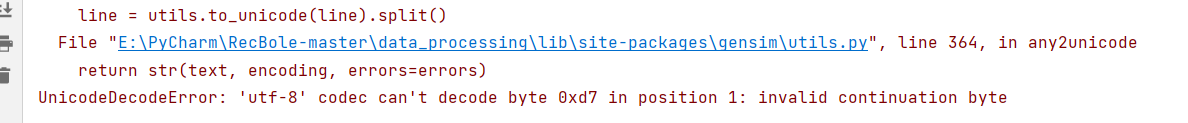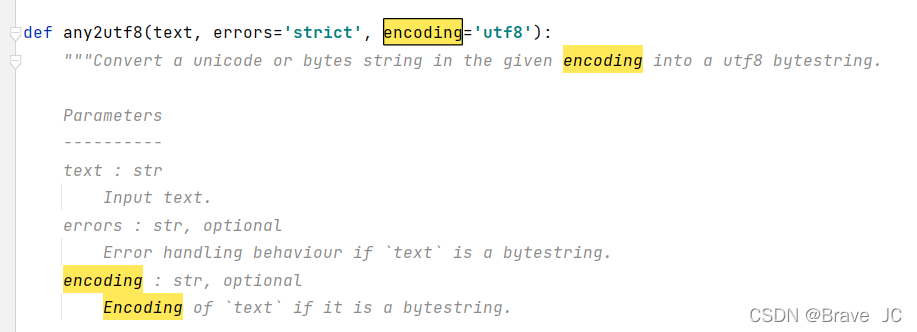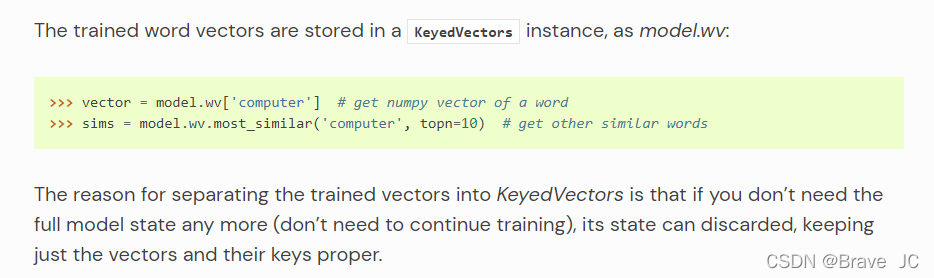# How to Solve word2vec Module Error: AttributeError & UnicodeDecodeError

1. Decode Issues
1. Error Messages: UnicodeDecodeError: ‘utf-8’ codec can’t decode byte 0xd7 in position 1
2. Error Codes:

``````Insert # -*- coding: utf8 -*-

"""Write out the processed data"""
for row in no_stop_review:
f.write(row[-1])
f.write("\n")
f.close()

if __name__ == '__main__':
segment_text = cut_words('audito_whole.csv')
review_text = remove_punctuation(segment_text)
write_review(review_text, 'stop_words.txt', 'no_stop.txt', 'stop.txt')

# Model Training Master Program
logging.basicConfig(format='%(asctime)s : %(levelname)s : %(message)s', level=logging.INFO)
sentences_1 = word2vec.LineSentence('no_stop.txt')
model_1 = word2vec.Word2Vec(sentences_1)

# model.wv.save_word2vec_format('test_01.model.txt', 'test_01.vocab.txt', binary=False)  # 保存模型，后面可直接调用
# model = word2vec.Word2Vec.load("test_01.model")  # Calling the model

# Calculate the list of related words for a word
a_1 = model_1.wv.most_similar(u"Kongjian", topn=20)
print(a_1)

# Calculate the correlation of two words
b_1 = model_1.wv.similarity(u"Kongjian", u"Houzuo")
print(b_1)
``````

3. Error parsing: this error means that the character encoded as 0xd7 cannot be parsed during decoding. This is because the encoding method is not specified when saving the text, so the GBK encoding may be used when saving the text
4. Solution: when writing out the data, point out that the coding format is UTF-8, as shown in the following figure

``````def write_review(review, stop_words_address, stop_address, no_stop_address):
"""Write out the processed data"""
with open(stop_address, 'a', encoding='utf-8') as f:
for row in review:
f.write(row[-1])
f.write("\n")
f.close()

with open(no_stop_address, 'a', encoding='utf-8') as f:
for row in no_stop_review:
f.write(row[-1])
f.write("\n")
f.close()
``````

5. Expansion – why did this mistake happen
first of all, we click the source code file of the error report, as shown in the following figure:open utils, and we find the following codeit can be seen that the encoding format set by word2vec is UTF-8. If it is not UTF-8 during decoding, errors = ‘strict’ will be triggered, and then UnicodeDecodeError will be reported

2. Attribute error
1 Error reporting: attributeerror: ‘word2vec’ object has no attribute ‘most_similar’
2. Wrong source code:

`````` # Model Training Master Program
logging.basicConfig(format='%(asctime)s : %(levelname)s : %(message)s', level=logging.INFO)
sentences_1 = word2vec.LineSentence('no_stop.txt')
model_1 = word2vec.Word2Vec(sentences_1)

# model.wv.save_word2vec_format('test_01.model.txt', 'test_01.vocab.txt', binary=False)  # 保存模型，后面可直接调用
# model = word2vec.Word2Vec.load("test_01.model")  # Calling the model

# Calculate the list of related words for a word
a_1 = model_1.most_similar(u"Kongjian", topn=20)
print(a_1)
``````

**3. Analysis of error reports: * * error reports refer to attribute errors because the source code structure has been updated in the new word2vec. See the official website for instructions as follows:**4. Solution: * * replace the called object as follows:

`````` # Model Training Master Program
logging.basicConfig(format='%(asctime)s : %(levelname)s : %(message)s', level=logging.INFO)
sentences_1 = word2vec.LineSentence('no_stop.txt')
model_1 = word2vec.Word2Vec(sentences_1)

# model.wv.save_word2vec_format('test_01.model.txt', 'test_01.vocab.txt', binary=False)  # 保存模型，后面可直接调用
# model = word2vec.Word2Vec.load("test_01.model")  # Calling the model

# Calculate the list of related words for a word
a_1 = model_1.wv.most_similar(u"Kongjian", topn=20)
print(a_1)
``````

5. Why is model_1.wv.most_similar(), I didn’t find the statement about WV definition in word2vec. Does anyone know?